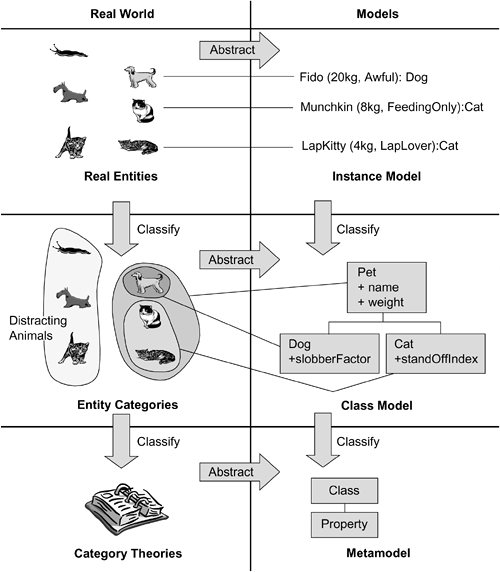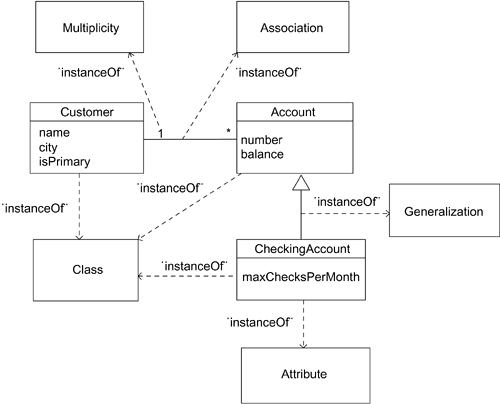# Metamodels

A metamodel is the result of a process of abstraction, classification, and generalization on the problem domain of the modeling language. The box that we commonly think of as a class is a concept in a modeling language, as are the other concepts we know from the UML, such as operations, activities, and states. Fundamentally, then, a metamodel is a model of the modeling language.

Figure 4-1 shows a small subset of the UML metamodel. ("Small" actually doesn't do it justice. There are 805 pages of text in the recently released UML 2.0 specification [Object Management Group, 2003], supported by goodness knows how many figures.) The classes in the metamodel capture the concepts Class, Property, Operation, and so forth that we use to build UML models. The metamodel also says that a Class is a subclass of Classifier, and a Class can have many Properties. The metamodel, then, formalizes our knowledge about what the UML is.

##### Figure 4-1. Excerpt from the UML metamodelNote that we're using the UML as an example. We could build a model of any arbitrary modeling language, and all of the same arguments would apply. We chose the UML as an example because, as the introduction to every UML-related academic paper since 1998 will tell you, it is the lingua franca of systems development.

Figure 4-2 shows the relationship between the metamodel and the pet model we introduced in Chapter 3.

##### Figure 4-2. Metamodel in relation to developer modelsThis sketch extends Figure 3-1 to show the relationship of the metamodel to the model. Whereas the types defined for the problem domain under study were Pet, Cat, and Dog, now that we shift down one level, the problem domain under study is the modeling language itself. The types in the language are Class, Property, Operation, Generalization, and so on the classes that appear in Figure 4-1.

The third row in Figure 4-2 represents the model beyond the model in other words, the metamodel. The metamodel is a model of the modeling language: a model of the UML.

Now, this is where it gets weird.

The instances of the class Class have values that are themselves classes from the original developer's model. This relationship is often informally called the instance-of relationship. The two best-known examples of the instance-of relationship are an object being an instance of a class and a row in a relational database being an instance of a table definition.

It's important to recognize that this instance-of relationship the one that is "meta" is different from the instance-of relationship that relates instances at the same level, such as that between Fido and the object pointed to by F1D0. This distinction is drawn out in (Atkinson and Kuhne).

Figure 4-3 shows how the excerpt from the analysis model of our banking system relates to the UML metamodel, which is represented by Multiplicity, Association, Class, and Generalization. We could perform the same exercise for the design model we introduced in Chapter 3. A metamodel, then, has types from another model as its instances.

##### Figure 4-3. Banking model as instances of metamodel classesWhen you're using your UML tool, you draw a rectangular class shape to denote a model element that has properties. That act creates an instance of the class Class with the name of the class you specify, and instances of the class Property that also have names that you specify. Similarly, when you use a line to connect class shapes, the line expresses the fact that there is an Association between one Class and another. (Your tool also captured other information, such as the location of the boxes on the screen, so that the model can be redrawn at a later date, but that doesn't concern us here.)

What you've done, in fact, is to use the UML modeling language to capture your model implicitly in the UML's (explicit) metamodel.MDA Distilled. Principles of Model-Driven Architecture
ISBN: B00866PUN2
EAN: N/A
Year: 2003
Pages: 134